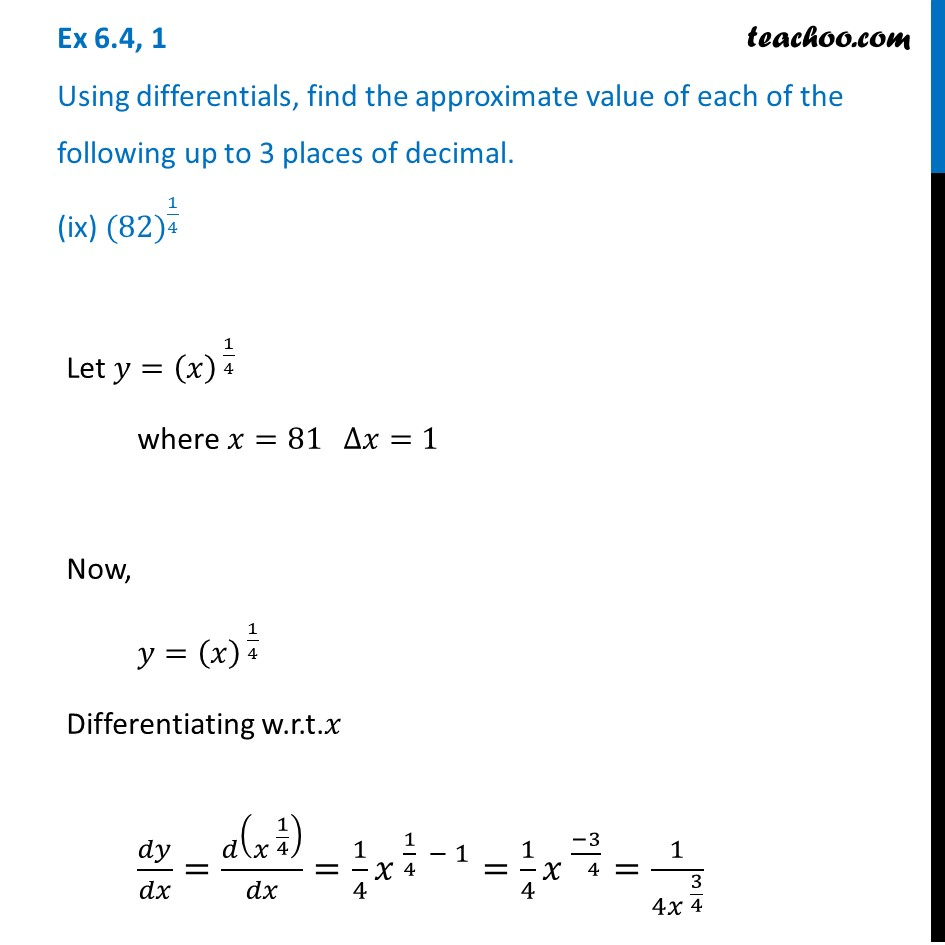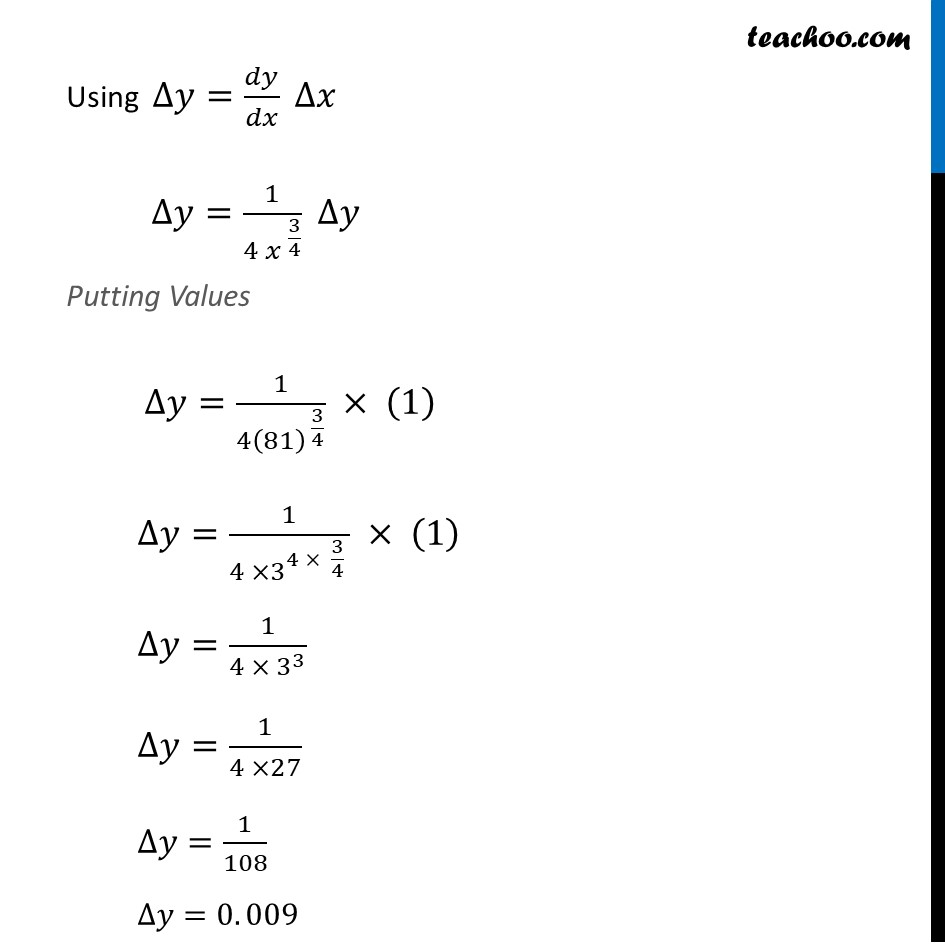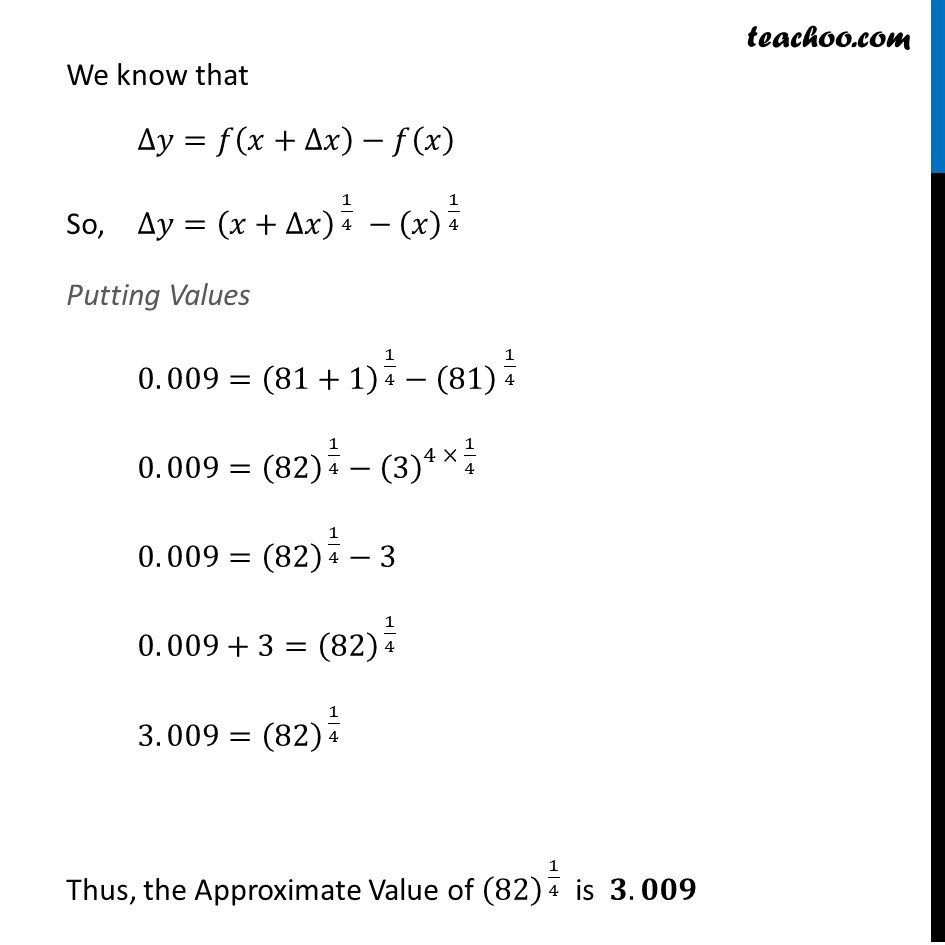Approximations (using Differentiation)

Chapter 6 Class 12 Application of Derivatives
Serial order wiseLearn in your speed, with individual attention - Teachoo Maths 1-on-1 Class

### Transcript

Question 1 Using differentials, find the approximate value of each of the following up to 3 places of decimal. (ix) 〖(82)〗^(1/4)Let 𝑦=(𝑥)^( 1/4) where 𝑥=81 ∆𝑥=1 Now, 𝑦=(𝑥)^( 1/4) Differentiating w.r.t.𝑥 𝑑𝑦/𝑑𝑥=𝑑(𝑥^( 1/4) )/𝑑𝑥=1/4 〖𝑥 〗^(1/4 − 1)=1/4 〖𝑥 〗^((−3)/( 4))=1/(4〖𝑥 〗^(3/4) ) Using ∆𝑦=𝑑𝑦/𝑑𝑥 ∆𝑥 ∆𝑦=1/(4〖 𝑥 〗^(3/4) ) ∆𝑦 Putting Values ∆𝑦=1/(4(81)^( 3/4) ) × (1) ∆𝑦=1/(4 ×3^(4 × 3/4 ) ) × (1) ∆𝑦=1/(4 × 3^3 ) ∆𝑦=1/(4 ×27) ∆𝑦=1/108 ∆𝑦=0. 009 We know that ∆𝑦=𝑓(𝑥+∆𝑥)−𝑓(𝑥) So, ∆𝑦=(𝑥+∆𝑥)^( 1/4) −(𝑥)^( 1/4) Putting Values 0. 009=(81+1)^( 1/4)−〖(81) 〗^(1/4) 0. 009=(82)^( 1/4)−(3)^(4 × 1/4 ) 0. 009=(82)^( 1/4)−3 0. 009+3=(82)^( 1/4) 3. 009=(82)^( 1/4) Thus, the Approximate Value of (82)^( 1/4) is 𝟑. 𝟎𝟎𝟗